Читать статью 'Equivalent thermal resistance of the road surface' в журнале Арктика и Антарктика на сайте nbpublish.comРусEngCnTranslate this page:
•••• Authors
••• Reviewing
••You can just close the window to don't translate

Back to contents

Arctic and Antarctica
Reference:

# Equivalent thermal resistance of the road surface

Galkin Aleksandr

ORCID: 0000-0002-5924-876Х

Doctor of Technical Science

Professor, Chief Researcher of the Melnikov Permafrost Institute SB RAS

677010, Russia, Yakutsk, ul. Merzlotnaya, 36, IMZ SO RAN. Laboratoriya geotermii kriolitozony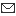afgalkin@yandex.ruДругие публикации этого автора

DOI:

10.7256/2453-8922.2022.3.38777

EDN:

HNHLEU

Review date:

15-09-2022

Publish date:

31-10-2022

Abstract: The design and construction of highways in the cryolithozone is associated with a number of difficulties, which are determined not only by geocryological and climatic operating conditions, but also by the complexity of the actual forecast of the thermal regime of road coverings and foundations. Many thermal calculations to substantiate technical solutions for the protection of highways in the cryolithozone from negative cryogenic phenomena are based on the determination and selection of a given thermal resistance of the structural layers of the pavement. The purpose of these studies was to assess the feasibility of using equivalent thermal resistance in modeling thermal processes and to determine the error in calculations that we make by replacing the layered pavement structure with an equivalent one.     Simple engineering dependences are obtained that allow us to determine the error in calculations when using equivalent thermal resistance. The calculation of the thermal resistance of the three-layer construction of the pavement is made. It is established that for a three-layer pavement structure, the error value in the calculation of thermal resistance is directly related to the degree of deviation of the values of the thermal conductivity coefficient of the materials of individual layers from each other. Moreover, the parameters of the inequality of thermal conductivity coefficients for individual structural layers when determining the minimum calculation error are functionally related to each other. The results of variant numerical calculations are presented in the form of 3D and 2D graphs, which allow us to visually assess the influence of the studied parameters on the relative error of calculating the thermal resistance of the pavement.

Keywords:

automobile road, cryolithozone, thermal mode, forecast, ratio, travel clothes, thermal resistance, equivalent layer, averaging, calculation error

Introduction.The design and construction of highways in the cryolithozone is associated with a number of difficulties, which are determined both by geocryological and climatic conditions of operation, and by the complexity of the actual forecast of the thermal regime of road coverings and foundations.

Calculation method.Using classical approaches to the definition of the concept of thermal resistance of a layered flat one-dimensional structure [16,17], the calculation formulas for finding the value of thermal resistance "R" of a pavement with a thickness of "?o" consisting of "n" layers of materials with a thickness of "?i" with a different coefficient of thermal conductivity "?i" can be written as follows.

For heterogeneous layered construction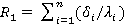(1)For an equivalent single-layer structure with an average value of the thermal conductivity coefficient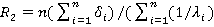(2)

The coefficient of thermal conductivity of a building material is usually determined experimentally or can be determined by calculation using known dependencies [18,19,20], which, as shown by the quantitative analysis performed in , give similar results sufficient for practical purposes.

Let's assume that the construction of the pavement consists of layers of equal thickness, but of different materials, i.e.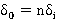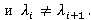then the parameter "R2/R1" is determined using equations (1) and (2) by the formula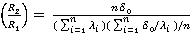=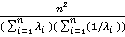(3)

The peculiarity of this expression is that it does not depend on the thickness of the individual layers and the total layer of the pavement, but only on the number of layers and the coefficient of thermal conductivity of the materials used in the pavement.Consider, for example, a simple three-layer construction of a road surface made of layers of equal thickness. In this case, expression (3) is converted to the form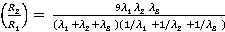(4)

For a two-layer construction, formula (3) will look even simpler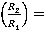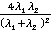(5)Next, as an example, we will consider the most typical three-layer construction of the pavement.

We introduce the parameters "k " and "m ", which determine the degree of inequality of the thermal conductivity coefficients of the materials of individual layers, i.e.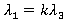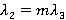in this case, the formula (4) is converted to the following form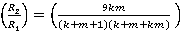(6)

The relative error that occurs when replacing a multilayer structure with an equivalent one-layer one is determined from the expression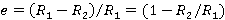(7)Multiplying the value obtained by formula (7) by 100%, we obtain a percentage relative error when calculating the thermal resistance of the pavement using the concept of an equivalent layer.

Let's check the validity of expressions (6) and (7). Substituting this value into expression (6) and assuming that k = m = 1 (that is, the construction consists of a homogeneous material), we obtain that the parameter (R 2 / R 1 ) = 1. That is, the error in calculating the thermal resistance when using an equivalent layer for a homogeneous structure, determined by the formula (7), is zero. Which was required to be proved and follows from the very formulation of the problem. In general, it was clear from simple physical considerations and follows from the formulation of the problem. After all, the value " k = m =1 " corresponds to the equality of the thermal conductivity coefficients of the first, second and third layers of pavement. Obviously, by dividing a homogeneous layer into three layers of equal thickness, we will not get any error in calculating the thermal resistance of the pavement.

Results and discussion.

To achieve the goal, variant calculations were carried out according to the above formulas, the results of which are presented in the form of 3D and 2D graphs in Figures 1-3. Figure 1 shows the change in the percentage error of determining the thermal resistance depending on the values of the parameters "k" and "m".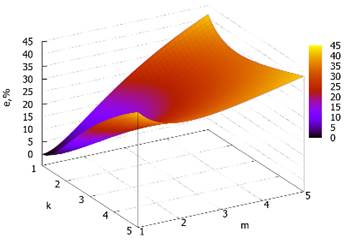Fig.1. Percentage error in determining thermal resistance depending on the values of the parameters "k" and "m".

As follows from the figure, there is an area in which the error is minimal. That is, the magnitude of the error is directly related to the degree of deviation of the values of the thermal conductivity coefficients of the materials of the individual layers of the pavement from each other (the values of the coefficients "m " and "k "). The more they differ from one, the greater the error. In the considered range of deviations of the values of the thermal conductivity coefficients, the error area of less than 10% (usually allowed in engineering practice) is not very large (highlighted in blue on the plane) and does not exceed the values of the inequality coefficients of more than two. This dependence is more clearly traced in Fig.2, where curves are shown that characterize the change in the percentage error depending on the parameter "k " for different values of the parameter "m ".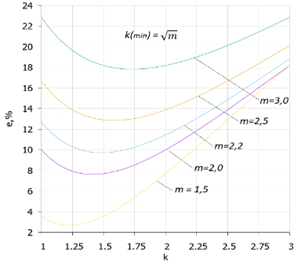Fig.2. Percentage relative error in determining thermal resistance depending on the value of the parameter "k" at different values of the parameter "m".

The figure clearly shows that there is a nonlinear relationship between the error of calculating the equivalent thermal resistance and the degree of inequality of the thermal conductivity coefficients of individual layers of pavement. At the same time, there is a clearly expressed minimum of the error allowed. To find it, it is necessary to investigate the function (7) at a minimum, i.e. solve a system of equations of the form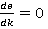and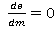, which allows us to find a functional relationship between the coefficients " m " and " k " , providing a condition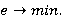for solving the system of equations, which is not difficult and leads us to the expression shown in Figure 2. That is, the minimum error is determined by the functional dependence between the parameters " m " and " k " of the simple form: m = k 2 .

Figure 3 shows curves characterizing the change in the error that occurs when determining the thermal resistance of the pavement, depending on the value of the coefficient of inequality of thermophysical properties " k " at different values of the parameter " m " with a color-highlighted area of permissible error in engineering practice.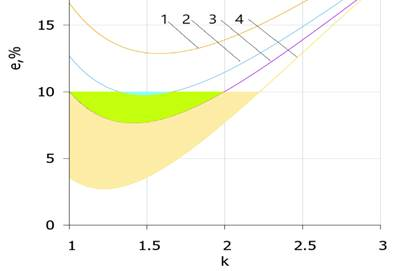Fig.3. The area of permissible error in determining the thermal resistance depending on the value of the parameter "k" at different values of the parameter "m": 1 – 2,5; 2 – 2,2; 3 – 2,0; 4 -1,5.Green, yellow and blue in the figure indicate the areas of permissible errors in the calculation of the desired value in engineering practice (e ? 10.0 %).

As can be seen from the graphs, this area significantly depends on the value of the parameter "m" and is small enough for values greater than "m" = 2.0.  And, at values of "m" ? 2.3, such a region is absent in the entire range of variation of the parameter "k". Considering that the range of changes in the thermophysical properties of building materials used in the structural layers of road coverings is not very large (with the exception of special heat-protective layers), it makes sense to determine the limit values of the range of variation of the coefficient of inequality "k" at different values of the parameter "m", which requires separate studies and is not considered in this paper.

Conclusion.  Simple engineering dependences are obtained to determine the error that occurs when calculating the thermal resistance of the pavement by replacing a layered structure with an equivalent single-layer one with an average coefficient of thermal conductivity. For clarity, an example of calculating the thermal resistance of a three-layer pavement structure is used. The concept of "coefficients of inequality of thermal conductivity" of materials of structural layers of pavement is introduced.  It is established that for a three-layer pavement structure, the error value in calculating thermal resistance is directly related to the degree of deviation of the values of the thermal conductivity coefficient of the materials of individual layers from each other. Moreover, the coefficients of inequality for individual layers in determining the permissible range of variation of the calculation error are functionally related to each other. Based on the study of the objective function of the calculation error, it is shown that the minimum error when using equivalent thermal resistance for three-layer pavement in thermal calculations is achieved with the ratio of inequality coefficients determined by the formula m = k 2 . The results of numerical calculations are presented in the form of 3D and 2D graphs, which allow us to visually assess the influence of the range of changes in the values of the thermal conductivity coefficients of materials of structural layers on the legality of using an equivalent single-layer pavement structure in the calculations of thermal resistance. The article can be useful for both design engineers of the road industry and researchers in the field of engineering geocryology and construction. In methodological terms, the article may be of interest to graduate students studying in various specialties of the direction 1.6. "Earth Sciences", as well as students studying in the specialty 08.02.05 "Construction and operation of highways and airfields".  Further research should be aimed at assessing the impact of the accepted theoretical model for calculating the thermal resistance of the pavement on the final result of the thermal calculation of the design values. For example, the estimated depth of thawing or freezing of the road base. It is also of interest to assess the error that occurs when designing road clothes with a special heat-protective structural layer.

References
 1 Vyalov S.S.(1978). Rheological foundations of frozen soil mechanics. M.: Vyssh. shkola. 2 Eppelbaum, L.V., Kutasov, I.M.(2019). Well drilling in permafrost regions: Dynamics of the thawed zone.Polar Research, 38, 3351. 3 Zhang, X., Feng, S.G., Chen, P.C. (2013). Thawing settlement risk of running pipeline in permafrost regions. Oil Gas Storage Transporation, 6, 365–369. 4 Galkin, A.F., Pankov, V.Yu. (2022). Thermal Protection of Roads in The Permafrost Zone. Journal of Applied Engineering Science, 20(2), 395-399, doi: 10.5937/jaes0-34379 5 Zhirkov, A.F., Zheleznyak, M.N., Shats, M.M., Sivtsev, M.A. (2021). Numerical modeling of changes in permafrost conditions of the airport Olekminsk. Surveying and subsoil use, 5 (115), 22-32. 6 Pankov, V.Yu., Burnasheva, S.G.(2020). Analysis of ways to protect roads from negative cryogenic processes. In. The best student article 2020.ICNS "Science and Enlightenment", 52-55. 7 Shats, M.M. (2011).The current state of the urban infrastructure of Yakutsk and ways to improve its reliability. Georisk, 2, 40–46. 8 Serikov, S.I., Shats, M.M. (2018). Frost cracking of soils and its role in the state of the surface and infrastructure of Yakutsk. Vestnik Permskogo natsional'nogo issledovatel'nogo politeknologii universiteta. Applied ecology. Urban studies,1, 56–69. doi: 10.15593/2409-5125/2018.01.04. 9 Shesternev, D.M., Litovko, A.V. (2018). Comprehensive studies to identify deformations on the Amur highway. In. Proceedings of the XIV All-Russian Scientific and Practical Conference and Exhibition "Prospects for the development of engineering surveys in construction in the Russian Federation". M .: Geomarket, 309-314. 10 Zheleznyak, M.N., Shesternev, D.M., Litovko, A.V.(2018). Problems of stability of roads in the permafrost zone. In. Proceedings of the XIV All-Russian Scientific and Practical Conference and Exhibition "Prospects for the development of engineering surveys in construction in the Russian Federation" M .: Geomarket, 223-227. 11 Kondratiev, V.G., Kondratiev, S.V. (2013). How to protect the federal highway "Amur" Chita-Khabarovsk from dangerous engineering and geocryological processes and phenomena. Engineering Geology, 5, 40-47. 12 Galkin, A.F., Kurta, I.V., Pankov, V.Yu., Potapov, A.V.(2020). Evaluation of the effectiveness of the use of layered structures of thermal protection in the construction of roads in cryolithozone. Energy safety and energy saving, 4, 24-28. 13 Bessonov, I.V., Zhukov, A.D., Bobrova, E.Yu., Govryakov, I.S., Gorbunova, E.A.(2022). Analysis of constructive solutions depending on the type of insulating materials in road surfaces in permafrostnye soils. Transport construction, 1, 14-17, doi: 10.18635/2071-2219-2020-4-24-28 14 Galkin, A.F., Zheleznyak, M.N., Zhirkov, A.F.(2021). Increasing the thermal stability of road clothes in cryolithozone. Building materials, 7, 26-31. 15 Galkin, A.F., Zheleznyak, M.N., Zhirkov, A.F.(2022). Criterion for the selection of building materials for thermal insulation layers of road clothes and foundations. Uspekhi sovremennogo estvoznaniya, 8, 108-113, doi.org/10.17513/use.37875 16 Isachenko, V.P., Osipova, V.A., Sukomel. A.S.(1981). Heat transfer.M.:Energoizdat. 17 Galkin, A.F.(2021). Thermal resistance of the heat-shielding layer of road clothing. Natural and technical sciences, 8, 97-99. 18 Dulnev, G.I. (1989).Thermal conductivity of wet porous materials. ITZH, 56(2), 261–291. 19 Odelevsky, V.I.(1951). Calculation of generalized conductivity of heterogeneous systems. ZHTF, 21(6), 667-685. 20 Dulnev, G.N., Zarichnyak, Yu.P. (1974).Thermal conductivity of mixtures and composite materials. L.: Energiya. 21 Galkin, А.F., Kurta, I.V., Pankov, V.Yu. (2020). Calculation of thermal conductivity coefficient of thermal insulation mixtures. IOPConf. Ser.: Mater. Sci. Eng. 918 (2020) 012009